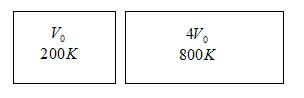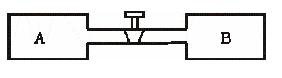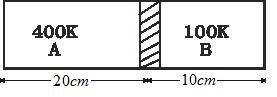# Assignment 1 on subjective type questions on Kinetic theory of gases

Question 1. Calculate the volume of 3 mole of an ideal gas at STP

Question 2. Calculate the mass of 4 cm3 of nitrogen at STP

Question 3.Equal masses of air are filled and sealed in two container ,one of volume V0 and other of the volume 4V0.If the first vessel is maintained at 200K and other at 800K.Find the ratio of the pressure in the two containersQuestion 4.An glass bulb of volume 390cc was sealed at a pressure of 2*10-3 mm of mercury at 25° C. Compute the number of the air molecules in the bulb .Avogadro number=6x1023 per mole, Density of mercury=13600Kg/m3
g=10m/s2

Question 5.A gas container has a walls that can bear maximum pressure of 2.2x106 pa.The container contains gas at 4x105 pa and 350K.If the container is steadily heated then neglecting change in volume, Calculate the temperature at which container will break?
Question 6. Density of an ideal gas at STP is 3x10-3 gm/cm3.Calculate the molecular weight of the gas

Question 7. A vessel of volume V=30 L contains ideal gas at temperature 0° C. After the portion of gas has been let out, the pressure in the vessel decreased by ΔP=.78 atm(Temp remaining constant).Find the mas of the gas released. The gas density under normal condition is ρ=1.3 g/L

Question 8. A vessel of volume V=20L contains a mixture of hydrogen and helium at T=20° C and Pressure P=2.0 atm.The mass of the mixture is equal to m=5g.Find the ratio of the mass of the hydrogen to that mass of the helium in the mixture. Given R=.082 L.atm.mol-1.K-1

Question 9. Find the rms speed of the hydrogen molecules in a sample of hydrogen gas at 250K .Find the temperature at which rms speed is three times the speed at 250K

Question 10.An ideal gas sample of .203 gm occupies 1000cm3 at STP.Calculate the RMS speed of the molecules

Question 11. The mean speed of the molecules of a hydrogen sample equals the mean speed of the molecules of helium sample. Calculate the ratio of temperature of hydrogen sample to the temperature of the helium sample.

Question 12. Find the ratio of the mean speed of the hydrogen molecule to the mean speed of the oxygen molecules in a sample containing mixture of two gases

Question 13. A vessel is partitioned by a fixed diathermic separator. Different ideal gases are filled in the two parts. The rms speed of the molecules in the left part equals the mean speed in the right part. Calculate the ratio of the mass of the molecule in the left part to the mass of the molecule in the right part?

Question 14.An air bubble of radius 3.00mm is formed at the bottom of the 4m deep river. Calculate the radius of the air bubble as it comes to the surface .Atmospheric pressure=105 Pa and density of the water=1000 kg/m3

Question 15. Air is pumped into the tubes of a cycle at 1.5 atm pressure and volume of tube at this pressure is .002 m3.When one of the tubes get punctured volume of tube reduces to .0004m3. Calculate the number of moles that leaked from the tube assuming that air is an ideal gas and temperature remains constant at 300K.

Question 16. A tank attached to an air compressor contains 30L of air at temperature of 40° C and gauge pressure 5*105 pa. What is the mass of the air and what volume would it occupy at normal atmosphere pressure

Question 17. Figure below shows two vessels A and B with rigid walls containing ideal gases. The pressure ,temperature and volume are PA,TA,V in vessel A and PB,TB,V in vessel B.Th. vessels are now connected through a small tube .Show that the pressure P and Temperature T satisfy
P/T=1/2[PA/TA + PB/TB]
when equilibrium is reachedQuestion 18. Figure shows a cylindrical tube of length 30 cm partitioned by a tight fitting seprator.The separator is weekly conducting and can freely slide along the tube. Ideal gases are filled in the two parts of the vessel. In the beginning, temp in two parts A and B are 400K and 100 K respectively. The separator slides to a momentary equilibrium position as shown in fig.Find the equilibrium position of the separator reached after the long timeQuestion 19. One mole of ideal gas undergoes a process
P=P0/(1+(V/V0)2)
Where P0 and V0 are constant. Find the temperature of gas when V=V0

Question 20.
Calculate the variation of atmospheric pressure with elevation of the earth atmosphere. Considering the temperature to be uniform throughout (which is not the actual case)

Solutions